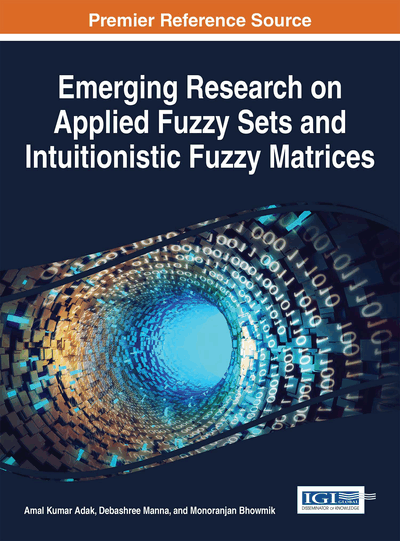# Non-Linear Intuitionistic Fuzzy Number and Its Application in Partial Differential Equation

Sankar Prasad Mondal (National Institute of Technology Agartala, India)
DOI: 10.4018/978-1-5225-0914-1.ch009
Available
\$37.50
No Current Special Offers

## Abstract

In this chapter the concept of non linear intuitionistic fuzzy number is addressed. Mainly the construction of non linear intuitionistic fuzzy number whose membership function is non linear function is taken here. Finally the number is taken with partial differential equation and solve the problem.
Chapter Preview
Top

## Introduction

### Fuzzy Sets and Intuitionistic Fuzzy Sets

Generalizations of fuzzy sets theory (see Zadeh (1965)) is considered to be one of Intuitionistic fuzzy set (IFS). Out of several higher-order fuzzy sets, IFS was first introduced by Atanassov (1983) have been found to be suitable to deal with unexplored areas. The fuzzy set considers only the degree of belongingness and non belongingness. Fuzzy set theory does not incorporate the degree of hesitation (i.e., degree of non-determinacy defined as, 1- sum of membership function and non-membership function. To handle such situations, Atanassov (1983) explored the concept of fuzzy set theory by intuitionistic fuzzy set (IFS) theory. The degree of acceptance in Fuzzy Sets is only considered, otherwise IFS is characterized by a membership function and a non-membership function so that the sum of both values is less than one (see Atanassov (1986)). Now-a-days, IFSs are being studied extensively and being used in different fields of Science and Technology.

### Fuzzy Differential Equation and Intuitionistic Fuzzy Differential Equation

The topic “fuzzy differential equation”(FDE) has been rapidly developing in recent years. The appliance of fuzzy differential equations is a inherent way to model dynamic systems under possibilistic uncertainty (Zadeh (2005)). The concept of the fuzzy derivative was first induced by Chang and Zadeh (1972). It was followed up by Dubois and Prade (1982). Other methods have been discussed by Puri and Ralescu (1983) and Goetschel and Voxman (1986). The concept of differential equations in a fuzzy environment was first formulated by Kaleva (1987). In fuzzy differential equation all derivative is deliberated as either Hukuhara or generalized derivatives. The Hukuhara differentiability has a imperfection (see Bede and Gal (2005)). The solution turns fuzzier as time goes by. Bede (2006) exhibited that a large class of BVPs has no solution if the Hukuhara derivative is used. To overcome this difficulty, the concept of a generalized derivative was developed (see Chalco-Cano and Roman-Flores (2008)) and fuzzy differential equations were discussed using this concept (see Bede et.al. (2007)). Khastan and Nieto (2010) found solutions for a large enough class of boundary value problems using the generalized derivative. Bede (2013) discussed the generalized differentiability for fuzzy valued functions. Pointedly the disadvantage of strongly generalized differentiability of a function in comparison H-differentiability is that, a fuzzy differential equation has no unique solution. Recently, Stefanini and Bede (2005) by the concept of generalization of the Hukuhara difference for compact convex set, introduced generalized Hukuhara differentiability (see Stefanini and Bede (2009)) for fuzzy valued function and they demonstrated that, this concept of differentiability have relationships with weakly generalized differentiability and strongly generalized differentiability. Recently Gasilov et. al. (2014) solve the fuzzy initial value problem by a new technique where Barros et. al. (2013) solve fuzzy differential equation via fuzzification of the derivative operator.

Intuitionistic FDE is very rare. Melliani and Chadli (2001) solve partial differential equation with intuitionistic fuzzy number. S. Abbasbandy, T. Allahviranloo (2002) discussed numerical Solution of fuzzy differential equations by Runge-Kutta and the Intuitionistic treatment. Lata and Kumar (2012) solve time-dependent intuitionistic fuzzy differential equation and its application to analyze the intuitionistic fuzzy reliability of industrial system. First order homogeneous ordinary differential equation with initial value as triangular intuitionistic fuzzy number is described by Mondal and Roy (2014). System of Differential Equation with Initial Value as Triangular Intuitionistic Fuzzy Number and its Application is solved by Mondal and Roy (2015).

## Complete Chapter List

Search this Book:
Reset